# Algebra and Trigonometry

Mathematics

## Quiz 4 : Exponential and Logarithmic FunctionsStudy FlashcardsLooking for Calculus Homework Help?

## Quiz 4 :Exponential and Logarithmic Functions

Question TypeEvaluate the function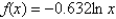at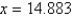. Round to 3 decimal places. (You may use your calculator.)
Free
Multiple Choice

E

Tags
Choose question tagLet Q represent a mass of radioactive radium (226Ra) (in grams), whose half-life is 1599 years. The quantity of radium present after t years is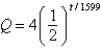Determine the quantity present after 200 years. Round to the nearest hundredth of a gram.
Free
Multiple Choice

A

Tags
Choose question tagFind the exact value of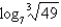without using a calculator.
Free
Multiple Choice

A

Tags
Choose question tagCondense the expression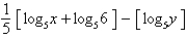to the logarithm of a single term.
Multiple Choice
Tags
Choose question tagSimplify the expression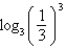.
Multiple Choice
Tags
Choose question tagRewrite the exponential equation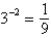in logarithmic form.
Multiple Choice
Tags
Choose question tagEvaluate the function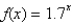at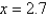. Round to 3 decimal places.
Multiple Choice
Tags
Choose question tagRewrite the logarithm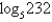in terms of the common logarithm (base 10).
Multiple Choice
Tags
Choose question tagRewrite the logarithm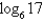in terms of the natural logarithm.
Multiple Choice
Tags
Choose question tagSolve the equation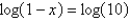for x using the One-to-One Property.
Multiple Choice
Tags
Choose question tagIdentify the value of the function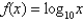at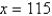. Round to 3 decimal places.
Multiple Choice
Tags
Choose question tagEvaluate the logarithm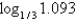using the change of base formula. Round to 3 decimal places.
Multiple Choice
Tags
Choose question tagWrite the exponential equation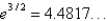in logarithmic form.
Multiple Choice
Tags
Choose question tagWhat is the value of the function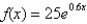at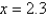? Round to 3 decimal places.
Multiple Choice
Tags
Choose question tagRewrite the logarithmic equation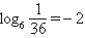in exponential form.
Multiple Choice
Tags
Choose question tagEvaluate the logarithm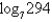using the change of base formula. Round to 3 decimal places.
Multiple Choice
Tags
Choose question tagCondense the expression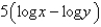to the logarithm of a single term.
Multiple Choice
Tags
Choose question tagIdentify the x-intercept of the function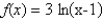.
Multiple Choice
Tags
Choose question tagCondense the expression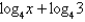to the logarithm of a single term.
Multiple ChoiceSimplify the expression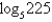.